# Dividing Mixed Numbers

Let's review how to divide fractions.

Here is an example: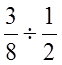We can use the KCF method. This stands for KEEP, CHANGE, FLIP.

Keep the first fraction:
Change from division to multiplication: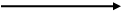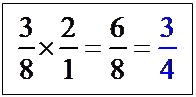Flip the second fraction over:

We can use these same steps when working with mixed numbers by adding one step in the beginning. That extra step is to change from mixed numbers to improper fractions.

Example #1:Step 1: Change to improper fractions.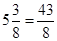and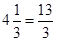Step 2: Write a new division problem with the improper fractions.Step 3: Use the KCF method to rewrite it as a multiplication problem.Step 4: Solve!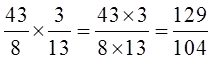Step 5: Rewrite as a mixed number.Here is another example.

Example #2:Step 1: Write the mixed numbers as improper fractions.Step 2: Rewrite the division problem using the improper fractions.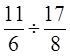Step 3: Use KCF.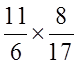Step 4: Multiply.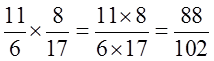Step 5: Simplify.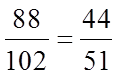So to divide mixed numbers you should change the fractions to improper fraction and the convert the problem to a multiplication question. Then you will be ready to solve.